## Excel FORECAST.ETS.CONFINT function

The FORECAST.ETS.CONFINT function calculates the confidence interval for the forecast value at the specified target date, which can be used along with the FORECAST.ETS function to grasp the forecast accuracy.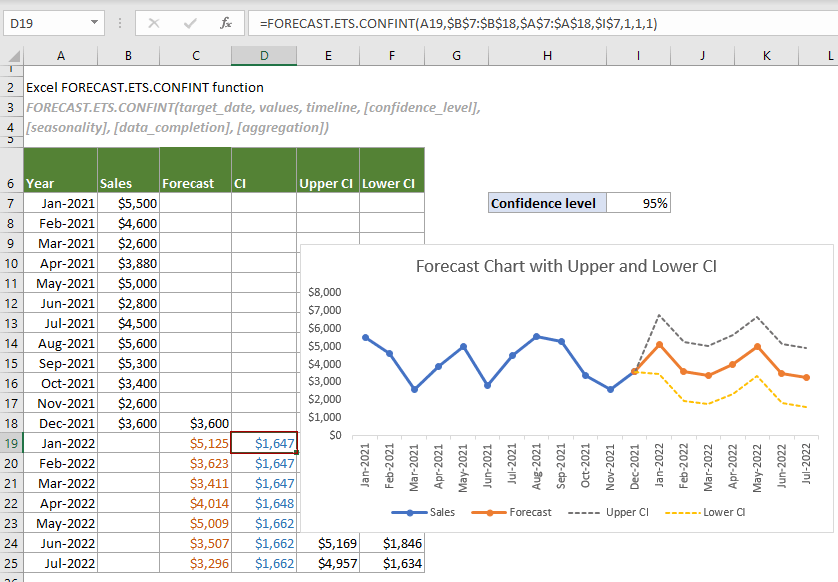Note: This FORECAST.ETS.CONFINT function is only available in Excel 2016 and later versions, and not available in Excel for the Web, iOS, or Android.

#### Syntax

FORECAST.ETS.CONFINT(target_date, values, timeline, [confidence_level], [seasonality], [data_completion], [aggregation])

#### Arguments

• Target_date (required): A date/time or a numeric value for which you want to predict a value;
• Values (required): The existing or historical known values for which you want to predict the next point (y values);
• Timeline (required): A range of date/time or numeric values corresponding to the “Values” (x values);
• Confidence_level (optional): A numeric value between 0 and 1 (exclusive) used to indicate a confidence level. If omitted, the default is 95%;
• Seasonality (optional): A numeric value used to define the length of the seasonal pattern. It can be:
0: No seasonality, which means that Excel will return a linear forecast;
1 or omitted (default value): Auto-detect, which means that Excel will automatically detect the seasonality and use positive, whole numbers for the length of the seasonal pattern;
N (an integer number): 2 ≦ N ≦ 8784 (the number of hours in a leap year), which means that Excel will use this specified number as the length of the seasonal pattern.
• Data_completion (optional): A numeric value specifies how to handle the missing points in the timeline. It can be:
0: Missing points will be treated as zeros;
1 or omitted: Missing points will be calculated as the average of the neighboring points.
• Aggregation (optional): A numeric value specifies which function will be used to aggregate several values with the same time stamp. The values and the corresponding functions are listed below.
 Numeric value Function 1 or omitted AVERAGE 2 COUNT 3 COUNTA 4 MAX 5 MEDIAN 6 MIN 7 SUM

#### Remarks

1. The #N/A error occurs if “values” and “timeline” arrays are not the same size;
2. The #NUM! error occurs when any of the following conditions is met:
-- 1 ≤ “confidence_level” ＜ 0;
-- All “timeline” values are the same;
-- A constant step cannot be identified in the provided timeline;
-- “seasonality” is not in the range (0-8784);
-- “data_completion” is any number other than 0 or 1;
-- “aggregation” is out of the valid range (1-7).
3. The #VALUE! error occurs when [confidence_level], target_date, [seasonality], [data_completion] or [aggregation] is nonnumeric.

#### Return value

It returns a numeric value.

#### Example

As shown in the table below, supposing you have applied the FORECAST.ETS function to predict the sales for January to July 2022 based on the existing monthly sales for 2021. Now you need to calculate the confidence intervals for the forecast values at the corresponding target dates and display the upper and lower confidence intervals in a forecast chart to help demonstrate the accuracy of the forecast values. Please do as follows to get it done.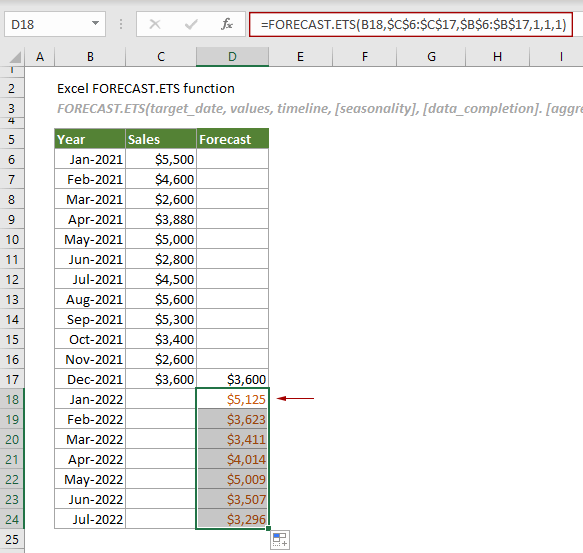1. Create three helper columns to locate the confidence intervals, upper confidence intervals, and the lower confidence intervals separately.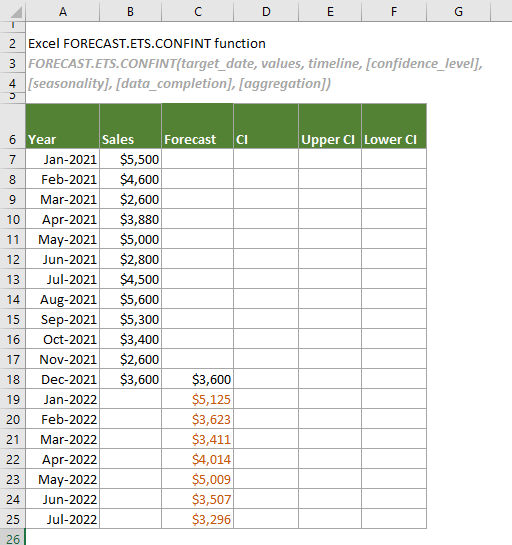2. Calculate the confidence intervals for the forecast values at the specified target dates.

In the confidence interval column (the CI column in this case), select the cell (D19) next to the first predicted value (C19) in the Forecast column, copy or enter the formula below and press the Enter key to get the result. Then select this result cell and drag its AutoFill Handle down to get the confidence intervals for other forecast values.

=FORECAST.ETS.CONFINT(A19,\$B\$7:\$B\$18,\$A\$7:\$A\$18,\$I\$7,1,1,1)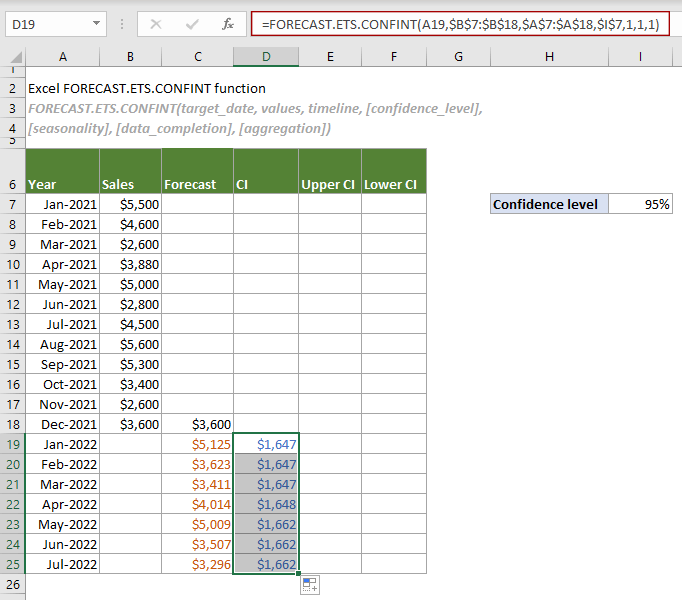Note: In the formula, \$I\$7 is the cell containing the specified confidence level. This reference cell can be replaced with 0.95 or 95%.

3. Calculate the upper confidence intervals.

In the Upper CI column, select a cell (E19 in this case) in the same row as the first forecast value, copy or enter the following formula and press Enter to get the result. Then select this result cell and drag its AutoFill Handle down to get other results.

=C19+D19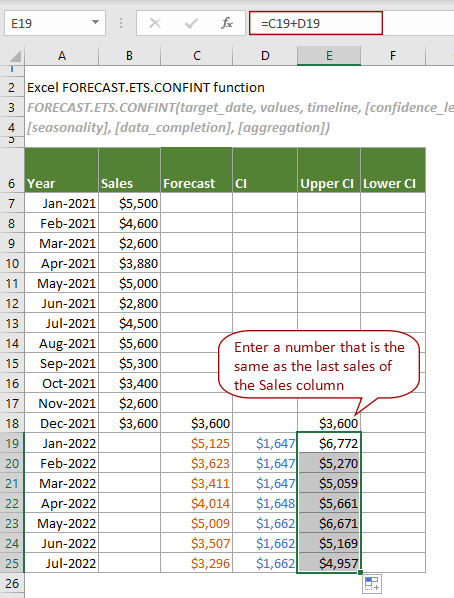4. Calculate the lower confidence intervals.

In the Lower CI column, do the same operation as step 3 to calculate the lower confidence intervals with the following formula.

=C19-D19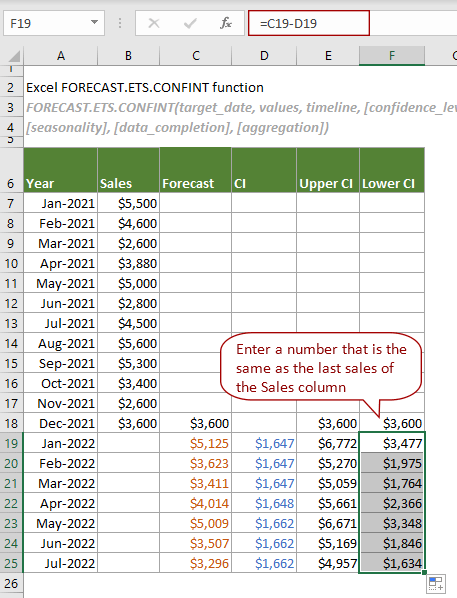Notes:

1) Upper confidence interval = forecast value + confidence interval;
2) Lower confidence interval = forecast value - confidence interval.

Now you can create a forecast chart with upper and lower confidence intervals as shown in the screenshot below.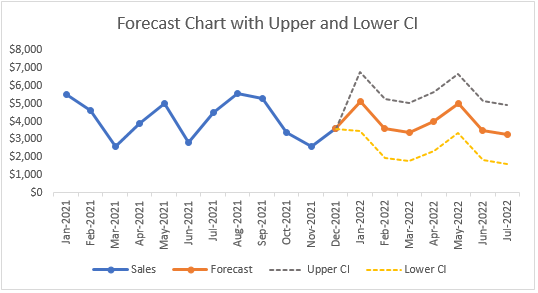#### Related Functions

Excel FORECAST function
The FORECAST function predicts a future value based on existing values by using the linear regression.

Excel FORECAST.ETS function
The FORECAST.ETS function uses the Exponential Smoothing (ETS) algorithm to predict a future value based on a series of existing values.

Excel FORECAST.ETS.SEASONALITY function
The FORECAST.ETS.SEASONALITY function returns the length of a seasonal pattern based on existing values and a timeline.

Excel FORECAST.ETS.STAT function
The FORECAST.ETS.STAT function returns a specified statistical value as a result of time series forecasting.

Excel FORECAST.LINEAR function
The FORECAST.LINEAR function predicts a future value based on existing values by using the linear regression.

### The Best Office Productivity Tools

#### Kutools for Excel - Helps You To Stand Out From Crowd

 Popular Features: Find, Highlight or Identify Duplicates  |  Delete Blank Rows  |  Combine Columns or Cells without Losing Data  |  Round without Formula ... Super VLookup: Multiple Criteria  |  Multiple Value  |  Across Multi-Sheets  |  Fuzzy Lookup... Adv. Drop-down List: Easy Drop Down List  |  Dependent Drop Down List  |  Multi-select Drop Down List... Column Manager: Add a Specific Number of Columns  |  Move Columns  |  Toggle Visibility Status of Hidden Columns  |  Compare Columns to Select Same & Different Cells ... Featured Features: Grid Focus  |  Design View  |  Big Formula Bar  |  Workbook & Sheet Manager | Resource Library (Auto Text)  |  Date Picker  |  Combine Worksheets  |  Encrypt/Decrypt Cells  |  Send Emails by List  |  Super Filter  |  Special Filter (filter bold/italic/strikethrough...) ... Top 15 Toolsets:  12 Text Tools (Add Text, Remove Characters ...)  |  50+ Chart Types (Gantt Chart ...)  |  40+ Practical Formulas (Calculate age based on birthday ...)  |  19 Insertion Tools (Insert QR Code, Insert Picture from Path ...)  |  12 Conversion Tools (Numbers to Words, Currency Conversion ...)  |  7 Merge & Split Tools (Advanced Combine Rows, Split Excel Cells ...)  |  ... and more

Kutools for Excel Boasts Over 300 Features, Ensuring That What You Need is Just A Click Away...#### Office Tab - Enable Tabbed Reading and Editing in Microsoft Office (include Excel)

• One second to switch between dozens of open documents!
• Reduce hundreds of mouse clicks for you every day, say goodbye to mouse hand.
• Increases your productivity by 50% when viewing and editing multiple documents.
• Brings Efficient Tabs to Office (include Excel), Just Like Chrome, Edge and Firefox.